# CH 610A/618A

Second Exam

Fall, 2002

Prof.N.L.Bauld

__________________________________________                                                                              Name (Last, First)

__________________________________________                                                                            Social Security Number

## Possible Points

Page/Pts/Points Earned

## Score

I.

19

2/8

3/11/

II.

23

4/12/

5/4/

6/7/

III.

15

7/10/

8/5/

IV.

17

9/7/

10/10/

V.

26

11/8/

12/10/

13/8/

Totals

100

### I. Acids and Bases in Organic Chemistry

A. Acid/Base Equilibria

1.     [6 pts]  Consider the following acid/base reactions and determine the positions of the equilibria using both a qualitative and a quantitative criterion in each case. In the first example, state both criteria which you are using. In both examples,  show precisely how they are applied.

a.[4 pts]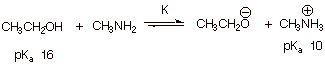(1). Qualitative Criterion: [1 pt]: The stronger acid is converted to the weaker acid. The lower pK­a corresponds to the stronger acid, so the methylammonium ion is converted to ethanol. That is, the equilibrium lies to the left.

(2). Quantitative Criterion [3 pts]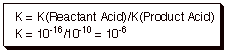b. [2 pts]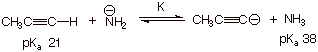(1). Apply Qualitative Criterion Stated Above: [1 pt]: Stronger acid is propyne; it is converted to the weaker acid ammonia; the reaction proceeds to the right.

(2). Apply Quantitative Criterion Stated Above: [1 pt]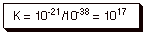B. Relative Acidities

1.    [2 pts] Write the equation for the acid dissociation of a general Bronsted acid, HA, in dilute aqueous solution (use the electron flow arrow formalism).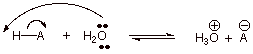2.    [3 pts] Cite two general effects which are considered to influence the order of relative acidities of a series of Bronsted acids in which only the nature of A is varied. Indicate in what sense (decrease or increase) they affect acidity and briefly explain why.

q      Anion Stability—The greater the stability (the lower the energy)of the anion, the more acidic is the Bronsted acid. That is because the anion is on the side of the equilibrium (the right side) which generates the hydronium ion.

q      Bond Dissociation Energy (D) of HA—the lower the value of D (i.e., the weaker the bond), the higher is the acidity of HA. This is because the formation of hydronium ion requires the dissociation of HA (or because the less the stability of HA, the less favored is the left hand side  of the equilibrium).

3.    [1 pt] Which of these two general effects is normally dominant?

q      Anion Stability.

4.    [1 pts] In what specific type of case is the alternate general effect dominant?

q      When A is varied down a column or Group of the periodic table.

6. [6 pts] For the following series of Bronsted acids, indicate the order of relative acidities (1 being the most acidic and 3 being the least acidic).  Explain the basis for this acidity order, including both a general effect and, if appropriate, a specific effect. Write the equation for the acid dissociation of the most acidic member of the group, using the electron flow formalism to show bond formation.[3 pts for each of a and b)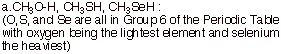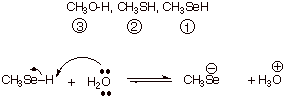q      This is a case of varying A down a Group of the periodic table. The bond dissociation energy effect is dominant here. The O-H bond is strongest and the Se-H bond is weakest.

q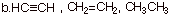q      Anion Stability is the General Effect.

q      The specific type of anion stability effect is hybridization.

q      The electron pair in the conjugate base of ethyne is in an sp AO, which is of lower energy than the sp2 AO of the ethene conjugate acid or the sp3 AO of the ethane conjugate acid.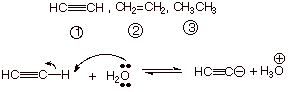### II. Stereochemistry

#### A. Number of Isomers

1.    [2 pt each = 4 pts] Indicate how many stereoisomers potentially exist for each of the following compounds and explain why this is the correct number.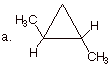q      There are three stereoisomers.

q      There are two stereocenters (the two front carbons), but they are equivalent (have all of the same substituents.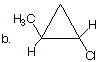q      Four stereoisomers.

q      Two, non-equivalent stereocenters.

b.    In the space above, draw the structures of the other stereoisomers involved in parts a and b above, and provide the terms which describe the relationships between the stereoisomers.

q      For part a, the  stereoisomers are: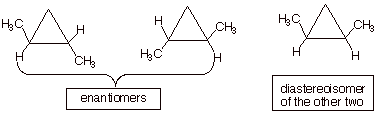q      For part b, the stereoisomers are: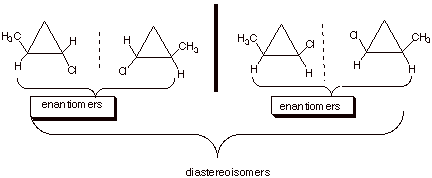B. Reactions Which Generate New Stereocenters

1.    Consider the following reaction of hydrogen chloride with 1-butene to give 2-chlorobutane: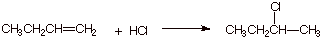a.     [4 pts] Provide three dimensional drawings of the two stereoisomers formed in this reaction and label them as R or S. Show explicitly the procedure you are using to derive the R, S designation.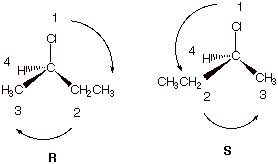q      If the illustration above is shown, no textual explanation is necessary.

b.    [2 pts] This reaction involves a carbocation intermediate, which reacts with chloride ion as a nucleophile. There are two possible transition states for reaction, each of which leads to a specific, unique product. Draw the structure of the product formed in each case, and label it as  R or S.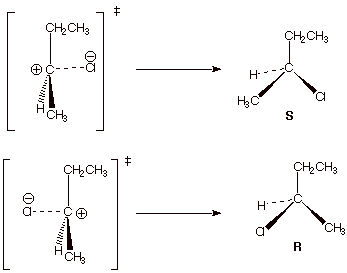c.     [2 pts] On the right side of the dashed line in the partial illustration shown below, draw the mirror image of the TS shown.

d.    [2 pts] What is the relationship between the two transition states for the addition of HCl to 1-butene? How does this relationship explain the relative amounts of the two products which are formed.

q      They are enantiomers (mirror images).

q      Since enantiomers have the same energy, these TS’s are equally favorable. Equal amounts of the R and S product are formed.

e.     [1 pts] What is the specific designation given to this kind of product mixture?

q      A racemic mixture(or racemate).

2.    Now suppose the reactant is (R)-3-chloro-1-butene, as shown below.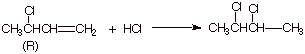a.     [2 pts] What are the R and S designations of the possible products (full IUPAC name).

q      (2R,3R)-dichlorobutane and (2R,3S) dichlorobutane).

q      [Note that since the stereocenters are equivalent, 2R,3S is the same as 2S,3R].

b.    [2 pts] What is the relationship between the two possible transition states shown below?

c.     They are diastereoisomeric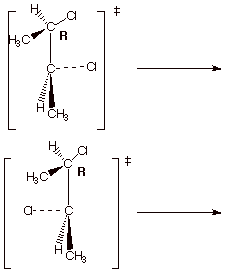### III. Alkenes: Nomenclature and Stability

A.  IUPAC Nomenclature

1. [3 pts] Provide the correct and complete IUPAC name for the following alkene, including any appropriate E/Z designation: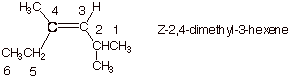2. [3 pts] Provide the correct structure of the alkene which has the IUPAC name cis-1-ethyl-3-(1,1-dimethylpropyl)cyclohexane.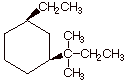B. Alkene Stability.

1.    [4 pts] The hydrogenation of both 1-butene and trans-2-butene gives the same product, butane. Illustrate, via an energy level diagram, the relative energies of these two alkenes, their energy difference in kcal/mol, and explain, using your diagram, how heats of hydrogenation reveal the exact magnitude of the difference in energies of these two alkenes.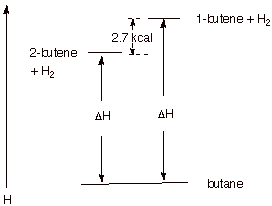q      Since both alkenes hydrogenate to the same product, any difference in their heats of hydrogenation must reflect energy differences in the alkenes (hydrogen is a common reactant, also). Since 1-butene has the higher heat of hydrogenation, it is the less stable (higher energy) alkene. The difference is ca. 2.7 kcal/mol (plus or minus 0.2 kcal acceptable).

2.    [2 pts] Explain why the difference in heats of hydrogenation of 1-pentene and trans-2-butene provides a good, approximate value for the difference in the thermodynamic energies of these non-isomeric alkenes.

q      Although the products of hydrogenation are different (pentane vs butane), the only bond which is broken in the case of either alkene is the pi bond. So differences in heats of hydrogenation of non-isomeric alkenes reflect, to a good approximation, differences in alkene stability.

C. Pi Bonding In Alkenes.

1. [3 pts] Illustrate by means of Newman projection structures, and also showing the relevant overlap of 2p orbitals in pi bonding, what happens when rotation around the C-C bond of cis-2-butene occurs to ultimately give trans-2-butene. Is this easy or difficult? How much of the pi bond remains at the 90 degree angle of rotation? Explain, using an illustration.

q      Difficult. The rotation around a pi bond diminishes the overlap between the 2p AO’s, which is maximum at zero degrees.

q      At the ninety degree dihedral angle, all pi bonding is lost (zero pi bond), because the net overlap is zero.

q      This is because the positive overlap which occurs in two volumes of space is exactly cancelled by the negative overlap in two other volumes of space.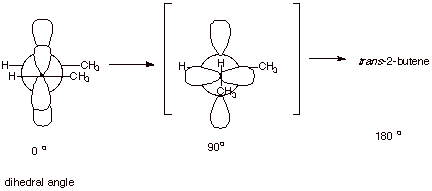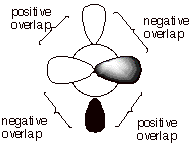IV. Reaction Mechanisms: General; Carbocation Intermediates

A.  Reaction Path Diagrams

1. Consider the following two step reaction: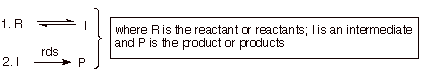a.     [5 pts] Sketch a reaction path diagram which realistically could represent such a reaction, indicating the position on the reaction coordinate of R, I, and P and any transition states (TS’s). Designate the TS’s as TS1 and TS2.

q      Note: A smooth curve would have been better, but that wasn’t possible with my software. The key points about the diagram are: (1) TS2 is higher than TS1, so that I returns to R more often than it goes on to P; (2) the product P is lower in energy than the reactant, R, otherwise the reaction wouldn’t go to completion.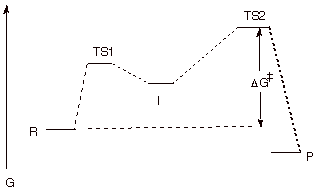b.    [1 pts] Indicate on the same diagram the energy quantity which controls the rate of the reaction.

q      This is shown on the diagram. It is the free energy difference between TS2 and R.

c.     [1 pt] What can be said about the effect upon the reaction rate of raising or lowering the energy of the intermediate (I) by a modest amount?

q      This would have no effect on the reaction rate, which depends only on the energy of TS2 and R.

d.    [3 pts] Consider the new mechanism shown below in which the first step is very slow (and is the slowest step of the reaction), but there is a significant amount of return of the intermediate to the reactant. Is the first step appropriately described as rate determining?  Explain why or why not, in detail.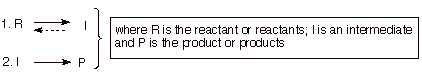q      No. A rds is a step the rate of which equals the rate of product formation. In this case the rate of the first step is faster than the rate of product formation, because some of the I returns to R, rather than going on to P. [It can be referred to as the “slow” step of the reaction]

C. Carbocation Intermediates

1. [2 pts] Which of the carbocations depicted below, the methyl carbocation or the ethyl carbocation is more thermodynamically stable and by how much (approximately, in solution)?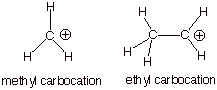q      The ethyl carbocation is the more stable cation by ca. 15 kcal/mol in solution [much more than this in the gas phase].

3.    [3 pts] Provide a resonance theoretical description of the resonance stabilization which exists in one of these carbocations. What specific sub-type of resonance effect is this?

q      This is called “hyperconjugation”.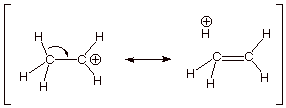4.    [2 pts] Provide the appropriate name for and a depiction of the other type of effect by which methyl or other alkyl groups stabilize a carbocation center.

q      The inductive effect.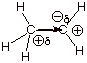V. Mechanism of H-Cl Additions to Alkenes

A. The Reaction Mechanism.

1. [3 pts] Write the detailed reaction mechanism for the addition of H-Cl to propene, employing all of the conventions of this course. Be certain to write each step of the mechanism on a separate, numbered line, to use electron flow arrows, and to designate any rate-determining steps or equilibrium steps.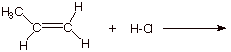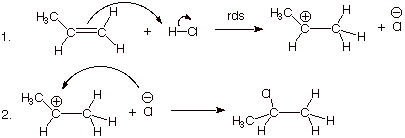2. [1 pts] If one of the steps of this mechanism is rate-determining, explain in energetic terms why it is this step in particular which is rate-determining and not the other step.

q      The first step is rate-determining because it involves breaking two bonds and forming only one. Therefore it is strongly endergonic; the second step involves forming one bond and breaking none, so it is highly exergonic and thus very fast.

3.    [4 pts] Draw a reaction path diagram for this reaction, specifying on your diagram the structures of I and P.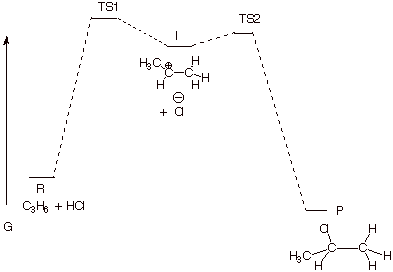q      A key point about this diagram is that TS2 must be lower in energy than TS1, so that I always goes on to P.

4.    [2 pts] What are the two major requirements for a step to be rate-determining? Point out on your diagram the way in which this reaction meets those requirements.

q      I must always go on to P (as stated above, TS2 lower in energy than TS1).

q      I must not build up in concentration. This means that the activation energy for step 2 must be very low.

B. Transition State Models.

1. [5 pts] Provide a resonance theoretical treatment of the transition state for the rds of this reaction, summarize this as a DL/PC (dotted line/partial charge) structure, and characterize the TS as specifically as you can (without yet applying the Hammond Principle).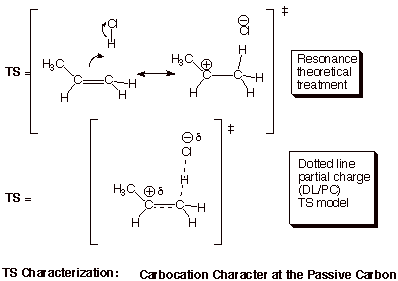2. [3 pts] State the Hammond Principle, and use it to further refine your TS model, explaining the steps in your logic.

q      The TS of a reaction step more closely resembles the higher energy partner (R or P) or that step.

q      Since, as stated earlier, the first step is highly endergonic (endothermic as well), the higher energy partner is the product of that step, which is the carbocation.

q      Therefore, the TS of this rds closely resembles the carbocation. We can say that the TS has “extensive (or highly developed) carbocation character at the passive carbon”.

C. Selectivity.

Consider, now, the hypothetical addition of H-Cl to propene in the opposite orientational sense, i.e., in which the product is 1-chloropropane.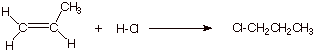1.    [4 pts] Modify the TS model you previously derived for the addition of HCl to propene to accommodate the different orientational sense. Compare the two models, side by side (Method of Competing TS’s), and provide a refined characterization of each TS model. Use this characterization to explain why the addition of HCl does not give 1-chloropropane.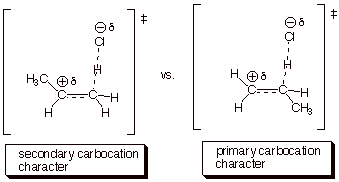q      The second TS is less favorable than the first, because primary carbocation character is less favorable than secondary carbocation character. The second TS would lead to 1-chloropropane.

2.    [2 pts] Explain how the Hammond Principle and its application to the rds of this reaction allow us to rationalize the selectivity of the HCl addition.

q      The Hammond Principle application indicated that there was extensive carbocation character. The greater the extent of carbocation character, the greater the sensitivity to the difference in stability of a secondary vs a primary carbocation.

q      The Hammond Principle therefore predicts a high degree of selectivity. (If there was little carbocation character, there would be relatively little selectivity).

3.    [1 pt] What type of selectivity is this (a formal name for the selectivity type)?

q      Regioselectivity (or Regiospecificity).

5. [1 pt] What is the symbolic mechanistic designation of this reaction?